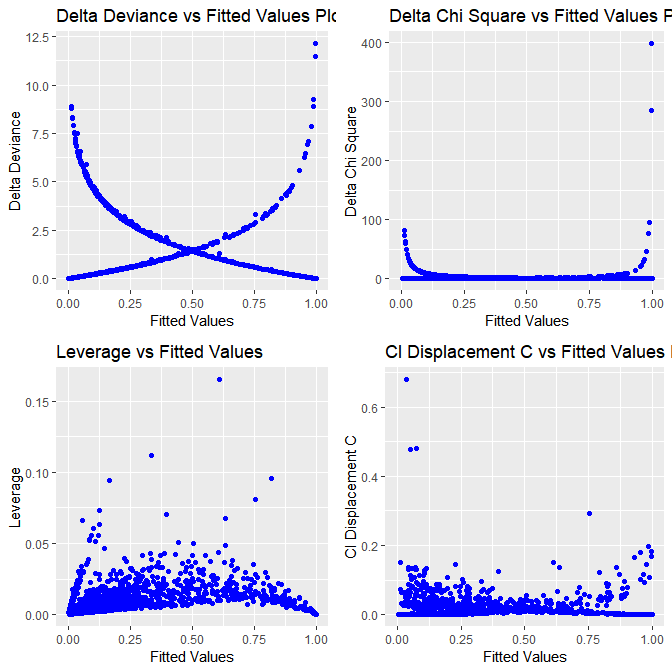A Short Introduction to the blorr Package

Introduction

The blorr package offers tools for building and validating binary logistic regression models. It is most suitable for beginner/intermediate R users and those who teach statistics using R. The API is very simple and most of the functions take either a data.frame/tibble or a model as input. blorr use consistent prefix blr_ for easy tab completion.

Installation

You can install blorr using:

The documentation of the package can be found at https://blorr.rsquaredacademy.com. This vignette gives a quick tour of the package.

Libraries

The following libraries are used in the examples in the vignette:

Data

To demonstrate the features of blorr, we will use the bank marketing data set. The data is related with direct marketing campaigns of a Portuguese banking institution. The marketing campaigns were based on phone calls. Often, more than one contact to the same client was required, in order to access if the product (bank term deposit) would be (‘yes’) or not (‘no’) subscribed. It contains a random sample (~4k) of the original data set which can be found at https://archive.ics.uci.edu/ml/datasets/bank+marketing.

Bivariate Analysis

Let us begin with careful bivariate analysis of each possible variable and the outcome variable. We will use information value and likelihood ratio chi square test for selecting the initial set of predictors for our model. The bivariate analysis is currently avialable for categorical predictors only.

blr_bivariate_analysis(bank_marketing, y, job, marital, education, default,
housing, loan, contact, poutcome)
#>                           Bivariate Analysis
#> ----------------------------------------------------------------------
#> Variable     Information Value    LR Chi Square    LR DF    LR p-value
#> ----------------------------------------------------------------------
#>    job             0.16              75.2690        11        0.0000
#>  marital           0.05              21.6821         2        0.0000
#> education          0.05              25.0466         3        0.0000
#>  default           0.02              6.0405          1        0.0140
#>  housing           0.16              72.2813         1        0.0000
#>   loan             0.06              26.6615         1        0.0000
#>  contact           0.31             124.3834         2        0.0000
#> poutcome           0.53             270.6450         3        0.0000
#> ----------------------------------------------------------------------

Weight of Evidence & Information Value

Weight of evidence (WoE) is used to assess the relative risk of di¤erent attributes for a characteristic and as a means to transform characteristics into variables. It is also a very useful tool for binning. The WoE for any group with average odds is zero. A negative WoE indicates that the proportion of defaults is higher for that attribute than the overall proportion and indicates higher risk.

The information value is used to rank order variables in terms of their predictive power. A high information value indicates a high ability to discriminate. Values for the information value will always be positive and may be above 3 when assessing highly predictive characteristics. Characteristics with information values less than 0:10 are typically viewed as weak, while values over 0.30 are sought after.

Plot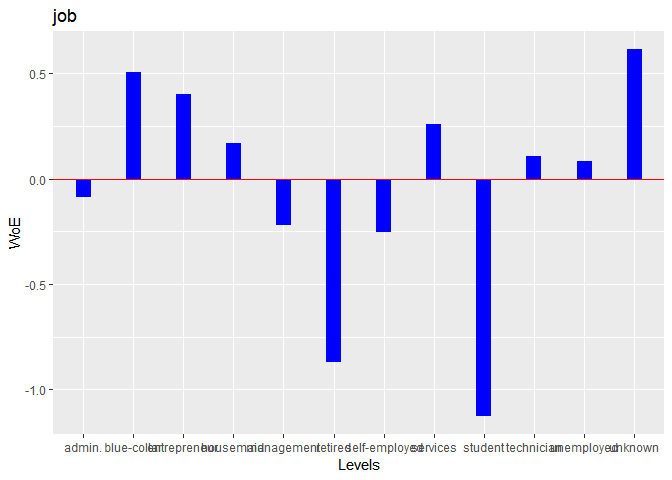Stepwise Selection

For the initial/ first cut model, all the independent variables are put into the model. Our goal is to include a limited number of independent variables (5-15) which are all significant, without sacrificing too much on the model performance. The rationale behind not-including too many variables is that the model would be over fitted and would become unstable when tested on the validation sample. The variable reduction is done using forward or backward or stepwise variable selection procedures. We will use blr_step_aic_both() to shortlist predictors for our model.

Model

Plot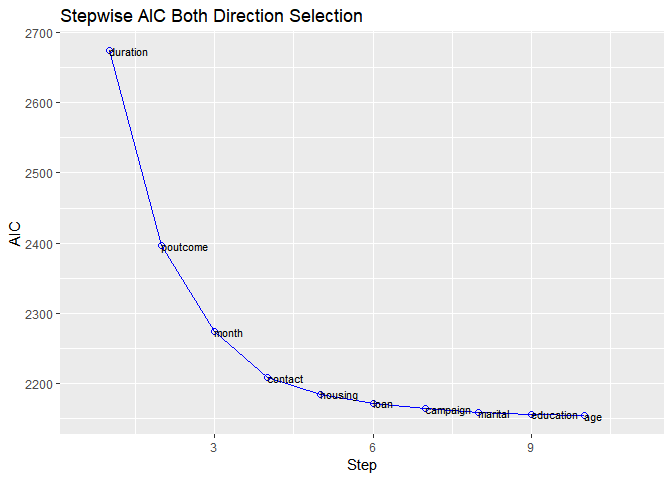Model Fit Statistics

Model fit statistics are available to assess how well the model fits the data and to compare two different models.The output includes likelihood ratio test, AIC, BIC and a host of pseudo r-squared measures. You can read more about pseudo r-squared at https://stats.idre.ucla.edu/other/mult-pkg/faq/general/faq-what-are-pseudo-r-squareds/.

Model Validation

Confusion Matrix

In the event of deciding a cut-off point on the probability scores of a logistic regression model, a confusion matrix is created corresponding to a particular cut-off. The observations with probability scores above the cut-off score are predicted to be events and those below the cut-off score, as non-events. The confusion matrix, a 2X2 table, then calculates the number of correctly classified and miss-classified observations.

The validity of a cut-off is measured using sensitivity, specificity and accuracy.

• Sensitivity: The % of correctly classified events out of all events = TP / (TP + FN)
• Specificity: The % of correctly classified non-events out of all non-events = TN / (TN + FP)
• Accuracy: The % of correctly classified observation over all observations = (TP + TN) / (TP + FP + TN + FN)

• True Positive (TP) : Events correctly classified as events.
• True Negative (TN) : Non-Events correctly classified as non-events.
• False Positive (FP): Non-events miss-classified as events.
• False Negative (FN): Events miss-classified as non-events.

For a standard logistic model, the higher is the cut-off, the lower will be the sensitivity and the higher would be the specificity. As the cut-off is decreased, sensitivity will go up, as then more events would be captured. Also, specificity will go down, as more non-events would miss-classified as events. Hence a trade-off is done based on the requirements. For example, if we are looking to capture as many events as possible, and we can afford to have miss-classified non-events, then a low cut-off is taken.

Hosmer Lemeshow Test

Hosmer and Lemeshow developed a goodness-of-fit test for logistic regression models with binary responses. The test involves dividing the data into approximately ten groups of roughly equal size based on the percentiles of the estimated probabilities. The observations are sorted in increasing order of their estimated probability of having an even outcome. The discrepancies between the observed and expected number of observations in these groups are summarized by the Pearson chi-square statistic, which is then compared to chi-square distribution with t degrees of freedom, where t is the number of groups minus 2. Lower values of Goodness-of-fit are preferred.

Gains Table & Lift Chart

A lift curve is a graphical representation of the % of cumulative events captured at a specific cut-off. The cut-off can be a particular decile or a percentile. Similar, to rank ordering procedure, the data is in descending order of the scores and is then grouped into deciles/percentiles. The cumulative number of observations and events are then computed for each decile/percentile. The lift curve is the created using the cumulative % population as the x-axis and the cumulative percentage of events as the y-axis.

Lift Chart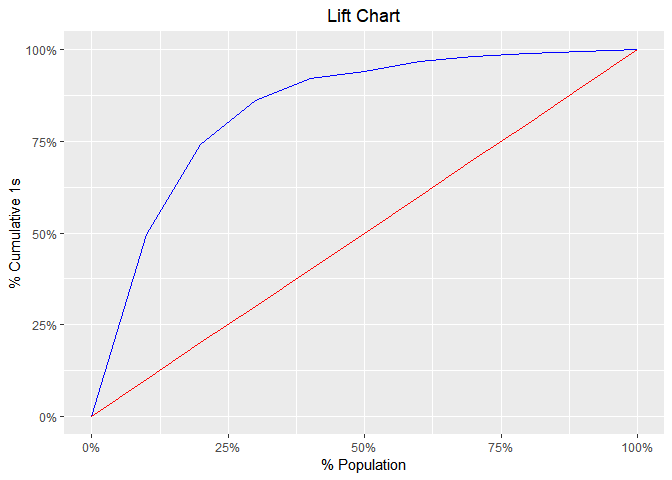ROC Curve

ROC curve is a graphical representation of the validity of cut-offs for a logistic regression model. The ROC curve is plotted using the sensitivity and specificity for all possible cut-offs, i.e., all the probability scores. The graph is plotted using sensitivity on the y-axis and 1-specificity on the x-axis. Any point on the ROC curve represents a sensitivity X (1-specificity) measure corresponding to a cut-off. The area under the ROC curve is used as a validation measure for the model – the bigger the area the better is the model.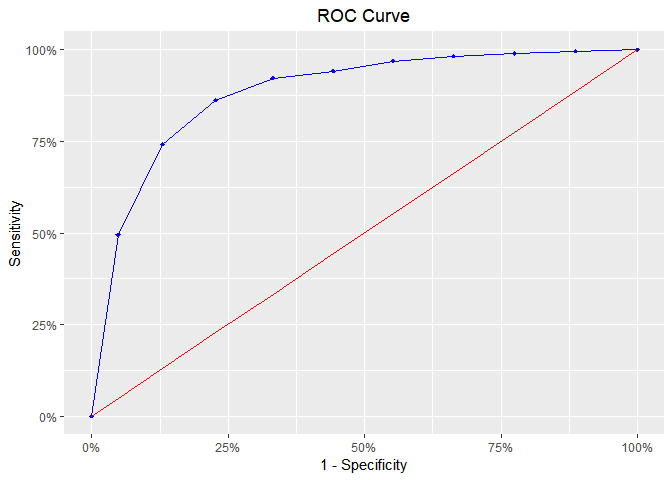KS Chart

The KS Statistic is again a measure of model efficiency, and it is created using the lift curve. The lift curve is created to plot % events. If we also plot % non-events on the same scale, with % population at x-axis, we would get another curve. The maximum distance between the lift curve for events and that for non-events is termed as KS. For a good model, KS should be big (>=0.3) and should occur as close to the event rate as possible.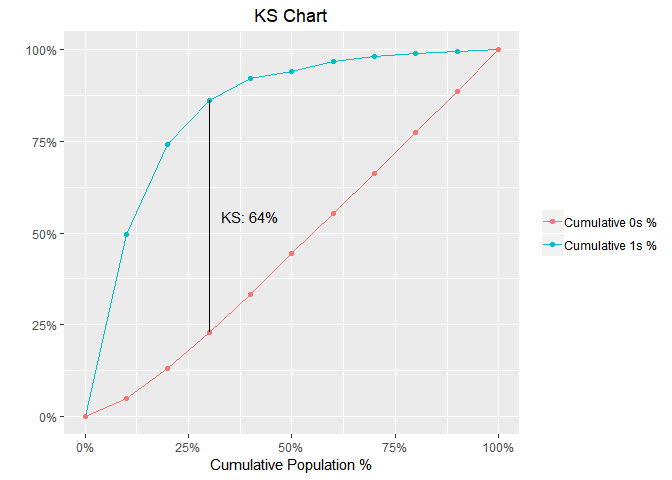Decile Lift Chart

The decile lift chart displays the lift over the global mean event rate for each decile. For a model with good discriminatory power, the top deciles should have an event/conversion rate greater than the global mean.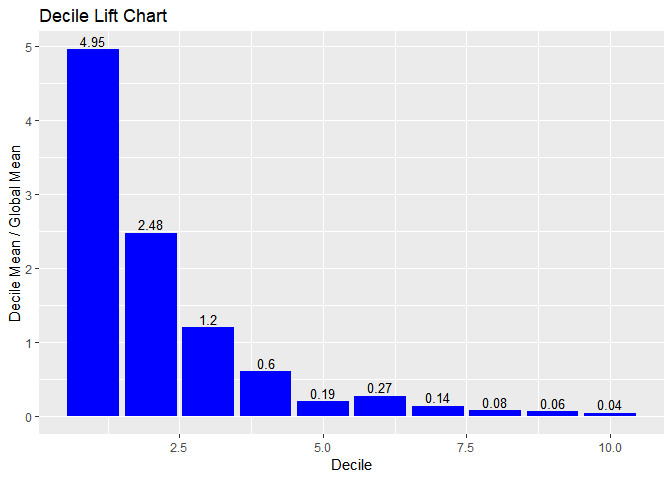Capture Rate by Decile

If the model has good discriminatory power, the top deciles should have a higher event/conversion rate compared to the bottom deciles.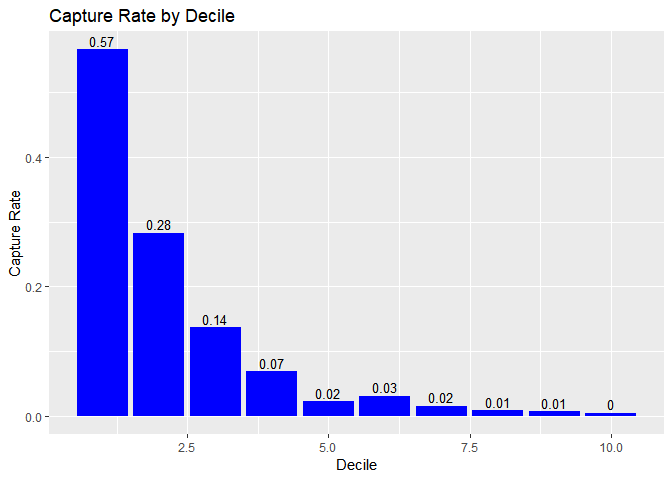Lorenz Curve

The Lorenz curve is a simple graphic device which illustrates the degree of inequality in the distribution of thevariable concerned. It is a visual representation of inequality used to measure the discriminatory power of the predictive model.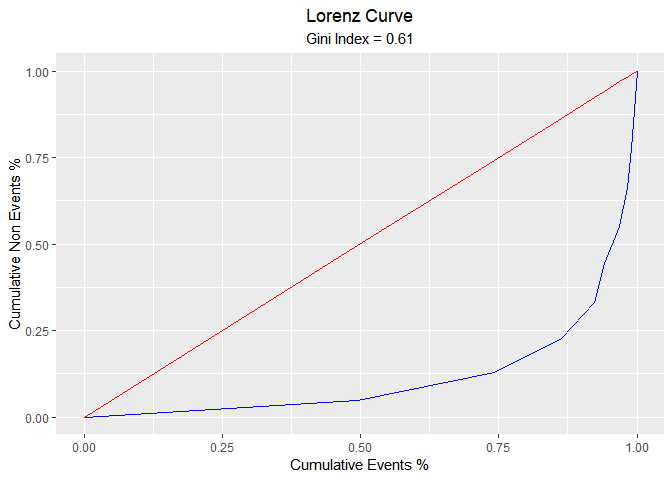Residual & Influence Diagnostics

blorr can generate 22 plots for residual, influence and leverage diagnostics.

Influence Diagnostics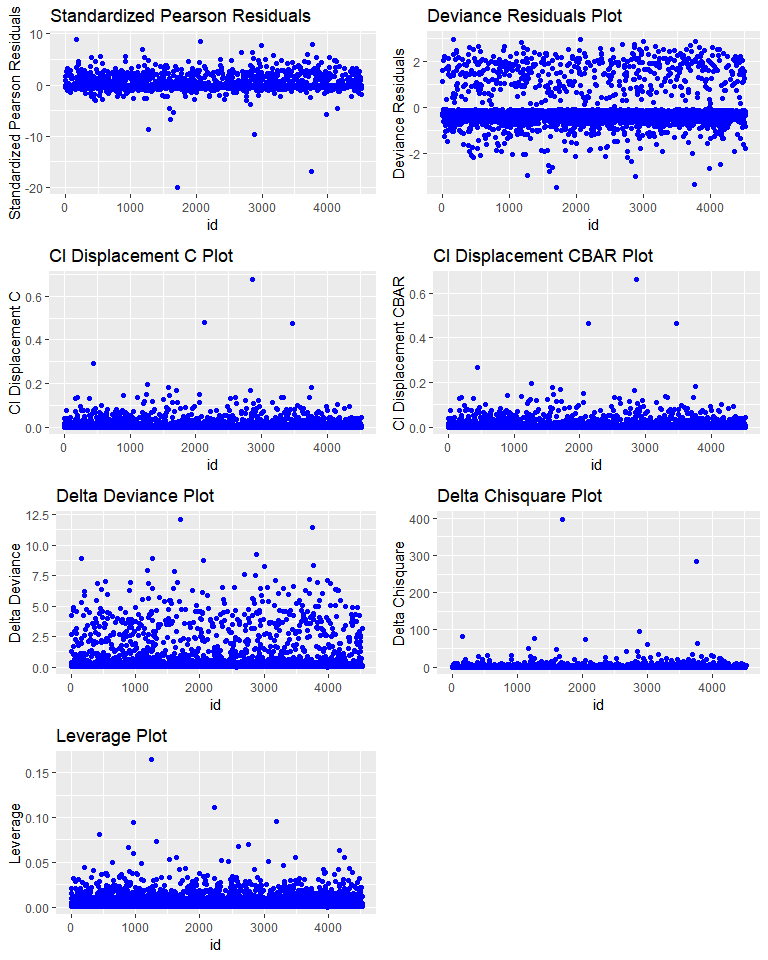Leverage Diagnostics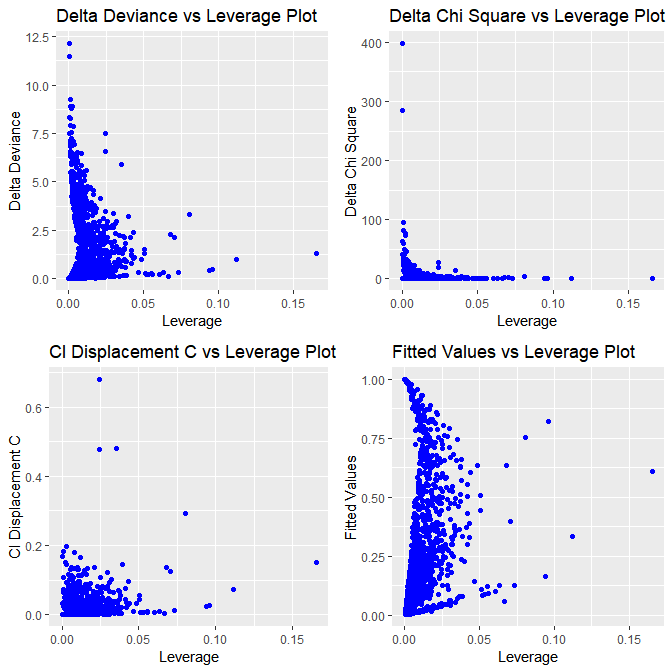Fitted Values Diagnostics